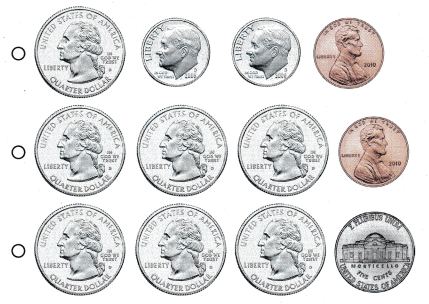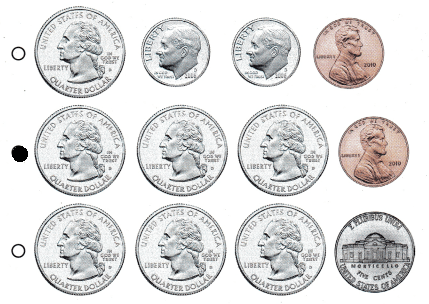Refer to our Texas Go Math Grade 1 Answer Key Pdf to score good marks in the exams. Test yourself by practicing the problems from Texas Go Math Grade 1 Lesson 9.3 Answer Key Quarters.

Essential Question
How can you find the total value of a group of coins?

Explore
Drawto show how Marisa trades.Explanation:
1 penny  = 1 cent.

For The Teacher

• Marisa knows a quarter has a value of 25 cents. She wants to trade some pennies for a quarter. How will Marisa trade?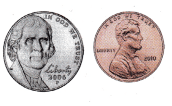1 nickel = 5 cents
1 penny = 1 cent
4 x 5 =20
1 x 5 = 5
20 + 5 = 25

Math Talk
How many nickels would trade for a quarter? Explain.
Explanation:
1 Nickel = 5 cents
5 x 5 = 25
so, 5 Nickels.

Problem Solving

Count. Write the total value.

Question 4.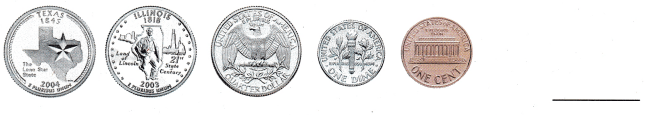Explanation:
1 Quarter dollar = 25¢
1 dime = 10¢
1 penny = 1¢
25 x 3 = 75
10 x 1 = 10
1 x 1 = 1
75 + 10 + 1 = 86

Question 5.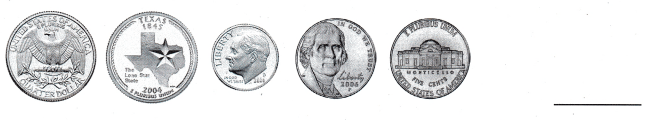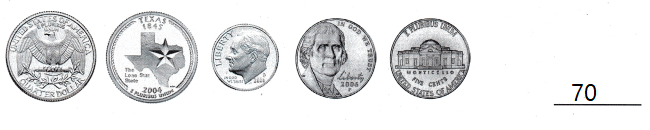Explanation:
1 Quarter dollar = 25¢
1 dime = 10¢
1 Nickel = 5¢
1 penny = 1¢
50 + 10 + 10 = 70

Question 6.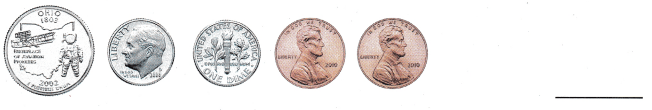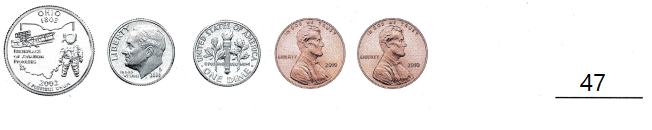Explanation:
1 Quarter dollar = 25¢
1 dime = 10¢
1 penny = 1¢
25 + 20 + 2 = 47

Question 7.
Matthew has 50¢ in his pocket. Draw the coins in his pocket.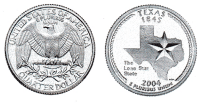Explanation:
1 Quarter dollar = 25¢
2 x 25 = 50¢

Question 8.
H.O.T. Multi-Step Keira has 75¢. Wyatt gives her a quarter. What is the total value of her coins?75 + 25 = 100= 1\$
Explanation:
Keira has 75¢.
Wyatt gives her a quarter.
100¢ is the total value of her coins

Use coins. Choose the correct answer.

Question 9.
Use Tools Count the coins. What is the total value?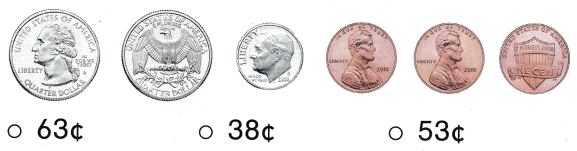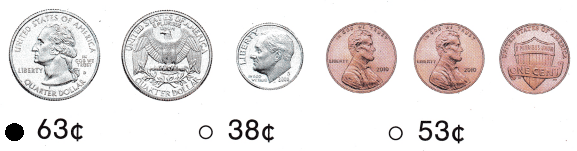Explanation:
1 Quarter dollar = 25¢
1 dime = 10¢
1 penny = 1¢
50 + 10 + 3= 63¢

Question 10.
Multi-Step Al has 4 coins. The value of the coins is 46¢. What coins does he have?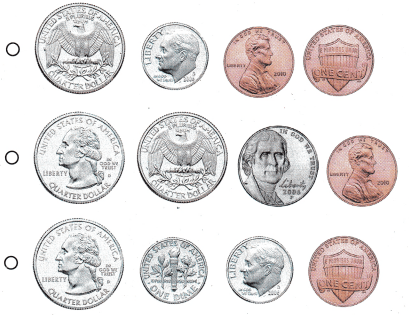Explanation:
1 Quarter dollar = 25¢
1 dime = 10¢
1 penny = 1¢
25 + 10 + 10+ 1= 46

Question 11.
Texas Test Prep What is the value of a quarter?
(A) 5¢
(B) 50¢
(C) 25¢
Explanation:
1 Quarter dollar = 25¢

Take Home Activity

• Have your child count groups of quarters, dimes, nickels, and pennies, with a maximum total value of 100¢.

### Texas Go Math Grade 1 Lesson 9.3 Homework and Practice Answer Key

Use coins. Write the total value.

Question 1.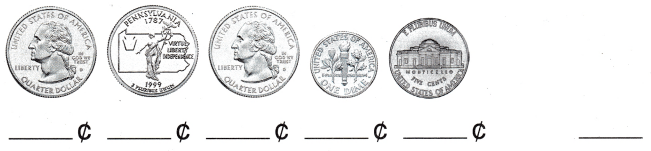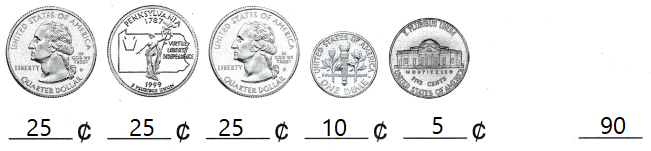Explanation:
1 Quarter dollar = 25¢
1 dime = 10¢
1 Nickel = 5¢
25 + 10 + 5 = 75¢

Question 2.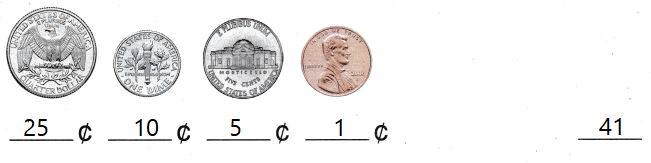Explanation:
1 Quarter dollar = 25¢
1 dime = 10¢
1 penny = 1¢
25 + 10 + 5 + 1 = 41

Draw and label coins to solve.

Question 3.
Leila paid 75¢ for an ice cream cone. Draw the coins Leila used to pay.Explanation:
1 Quarter dollar = 25¢
25 x 3 = 75¢

Texas Test Prep

Lesson Check

Question 4.
Jill finds these coins in her pocket. What is the total value of the coins?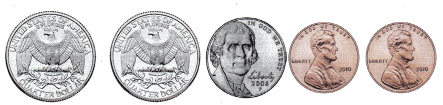(A) 57¢
(B) 62¢
(C) 27¢
Explanation:
1 Quarter dollar = 25¢
1 Nickel = 5¢
1 penny = 1¢
50 + 5 + 2 = 57¢

Question 5.
Count the coins. What is the total value?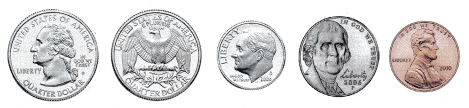(A) 71¢
(B) 61¢
(C) 66¢
Explanation:
1 Quarter dollar = 25¢
1 dime = 10¢
1 penny = 1¢
1 Nickel = 5¢
50 + 10 + 5 + 1 = 66¢

Question 6.
Multi-Step Tom saves money to buy a game. He puts 4 coins ¡n his bank. The value of the coins is 76¢. What coins does he have?